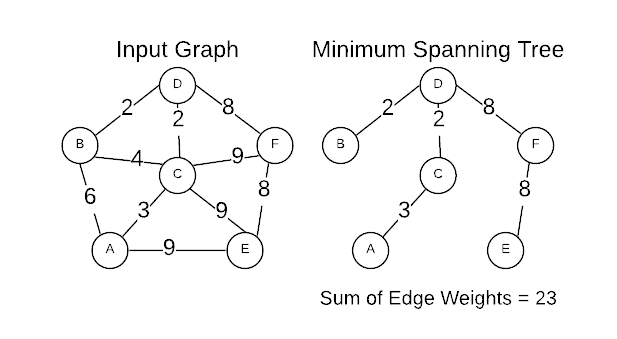# Minimum Spanning Tree (MST)

Prerequisite: Graph Concepts

A Minimum Spanning Tree (MST) is the cheapest set of edges that connect graph vertices together. For instance, servers on a network or destinations on a road map. Stated differently, an MST is the sub-set of edges with the minimum possible sum of edge weights. A MST must satisfy two properties.

1. Does not contain a cycle
2. A path exists between every pair of vertices

Based on the definition of a MST, the following facts logically apply:

• All connected graphs have at least one MST
• There are often multiple valid MSTs for a given graph
• An MST has $n-1$ edges.

The image below is a graphical representation of an input graph and its MST.This section explore algorithms for efficiently identifying the minimum spanning tree for a given graph.

## Applications

• Find the minimal connected sub-routes of a network (e.g. electrical grid, computer network) that will make it fully connected for the minimum cost
• Defining a taxonomy
• With a slight modification, Kruskal’s algorithm can be used for single-link clustering.

## Source Code

Full Repo

Relevant Directories: